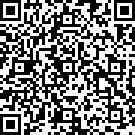在使用putimage加载出来的图片上使用outtextxy输出字符串失败

0

void printall（）
{
putimage(0, 0, &playlist);///
int position_x = 476;//“序号”横坐标
int position_y = 92;//“序号”纵坐标
setbkmode(TRANSPARENT);//设置字体背景为透明
settextcolor(COLORREF(RGB(0, 0, 0)));//设置字体颜色为黑色
settextstyle(20, 0, _T("楷体"));//设置字体大小20，格式楷体
outtextxy(position_x, position_y, _T("序号 初始时间 截止时间 日程内容 状态"));//显示表格第一行表头
position_y += 20;//向下推一行

if (!p)
{
//printf("歌库空空如也，快去添加吧~~\n");
outtextxy(556, position_y, "歌库空空如也，快去添加吧~~\n");

return;
}
for (p = HEAD; p; p = p->next)
{
if (p->song_id != -842150451)
outtextxy(556, position_y, p->name);
outtextxy(676, position_y, p->singer);
outtextxy(800, position_y, p->pinyin);
position_y += 20;//向下推一行
}
}

1 答案
0

1059085 (C语言革命0)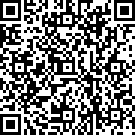8086730 (C语言革命1)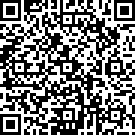2861971 (C语言革命2)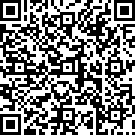19430220 (C语言革命6)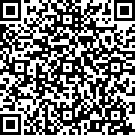46612969 (C语言革命7)